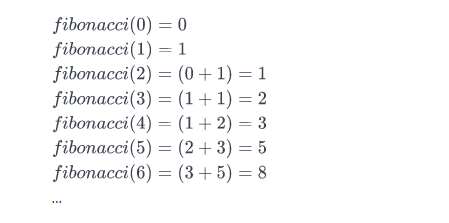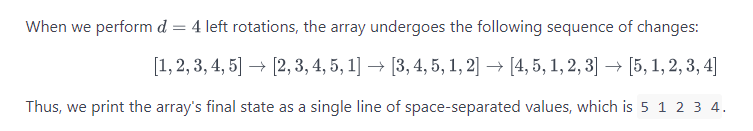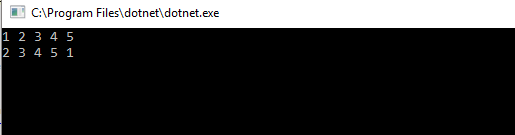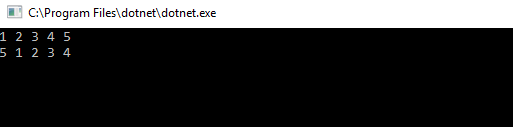## Consume php backend nested array on a C# Application – Json.NET

Lets assume you got a MYSQL database that contains data for your application and you want to consume this information on a C# Application in order to handle it on a client.

For the sake of this example the following table will be parsed

As depicted the following information is returned from the api:

• the state of the response (succeeded or not)
• a message that will show the response result
• the actual result that in this case is a list of trips

Each trip contains id, fromlocation, tolocation, date and time properties that have to be returned to the application.

The php code that was used to create the data from the database is the following:

So in order to parse this json result we will need a plugin like Newtonsoft.Json

The class that will be used to encapsulate the response would be the following:

And then we will consume the json response with the following code in C#. In this example data from the API came from a POST request on a web Form.

## Find the Nth Fibonacci Number – C# Code

The Fibonacci sequence begins with Fibonacci(0) = 0  and Fibonacci(1)=1  as its respective first and second terms. After these first two elements, each subsequent element is equal to the sum of the previous two elements.

Here is the basic information you need to calculate Fibonacci(n):

• Fibonacci(0) = 0
• Fibonacci(1) = 1
• Fibonacci(n) = Fibonacci(n-1) + Fibonacci(n-2)

Given n, complete the fibonacci function so it returns Fibonacci(n).

Input Format

Nth number

Output Format

Fibonacci() Nth number.

Sample Input

Sample Output

Explanation

Consider the Fibonacci sequence:We want to know the value of Fibonacci(3). If we look at the sequence above,Fibonacci(3)  evaluates to 2. Thus, we print 2 as our answer.

## Array Left Rotation by D – C# Code

left rotation operation on an array of size n shifts each of the array’s elements 1 unit to the left. For example, if 2 left rotations are performed on array [1,2,3,4,5], then the array would become  [3,4,5,1,2].

Given an array of  n integers and a number, d , perform d left rotations on the array. Then print the updated array as a single line of space-separated integers.

Input Format

The first line contains two space-separated integers denoting the respective values of  (the number of integers) and  (the number of left rotations you must perform).
The second line contains  space-separated integers describing the respective elements of the array’s initial state.

Output Format

Print a single line of  space-separated integers denoting the final state of the array after performing  left rotations.

Sample Input

Sample Output

ExplanationCode

We can see the results for 1 and 4 rotations as follows: